• Slides: 28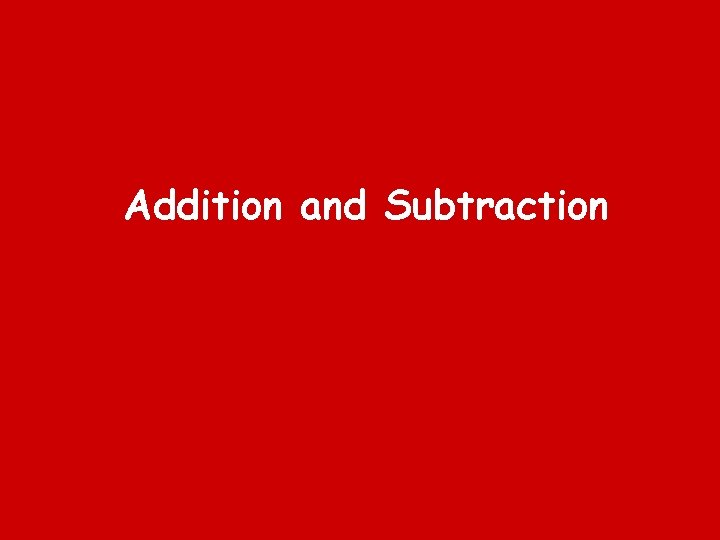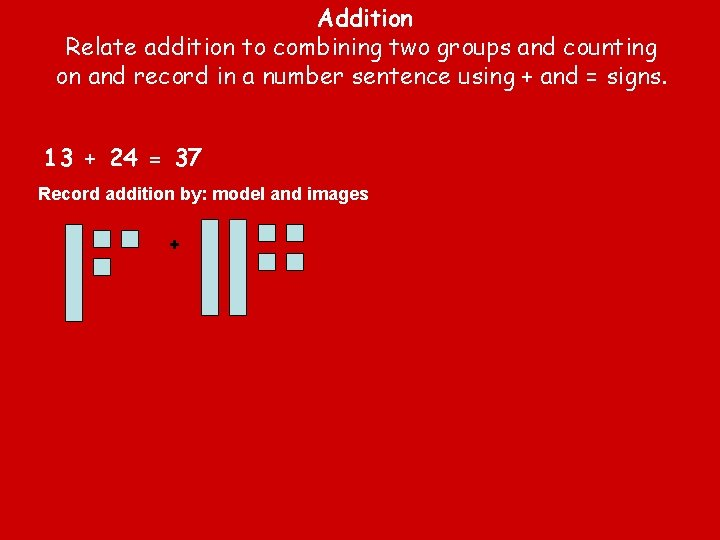Addition Relate addition to combining two groups and counting on and record in a number sentence using + and = signs. 13 + 24 = 37 Record addition by: model and images +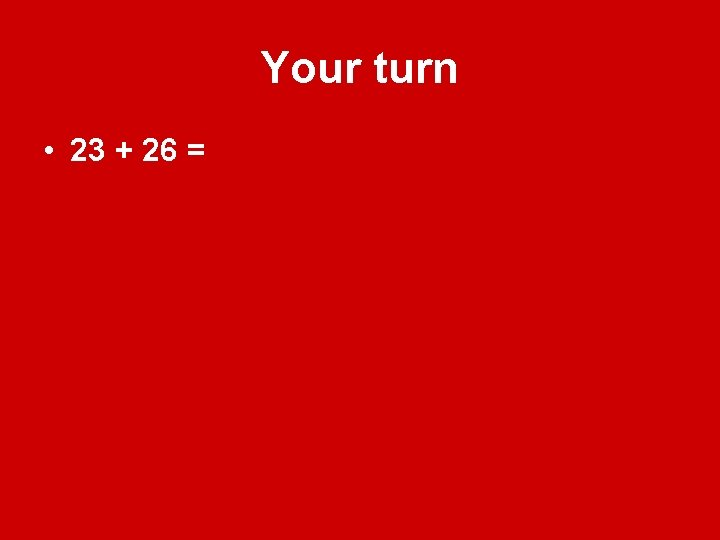Your turn • 23 + 26 =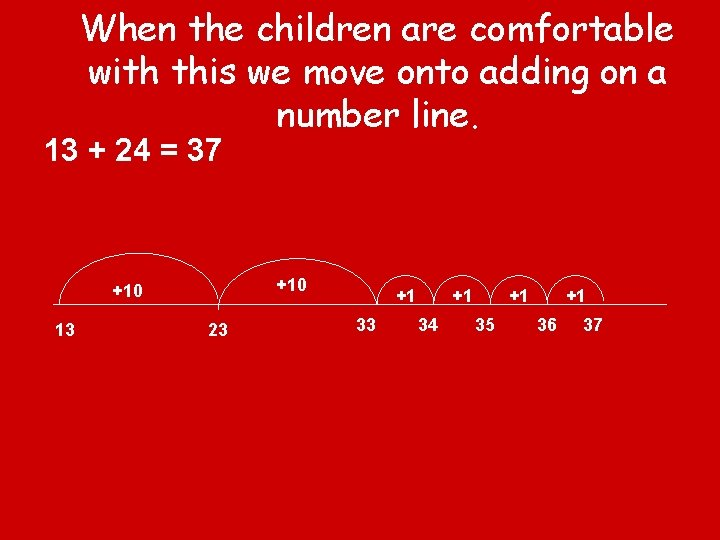When the children are comfortable with this we move onto adding on a number line. 13 + 24 = 37 +10 13 23 +1 34 +1 35 +1 36 37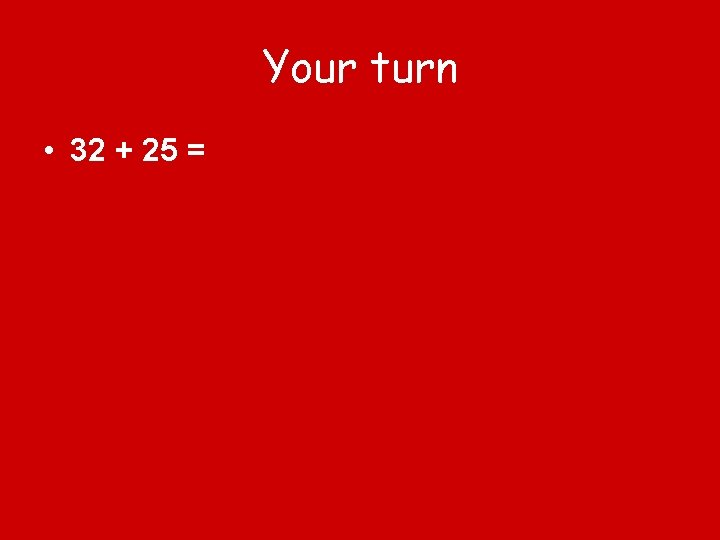Your turn • 32 + 25 =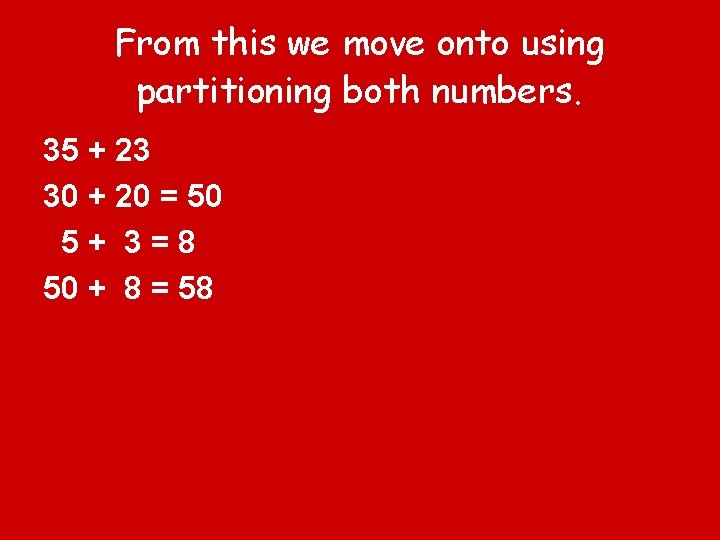From this we move onto using partitioning both numbers. 35 + 23 30 + 20 = 50 5+ 3=8 50 + 8 = 58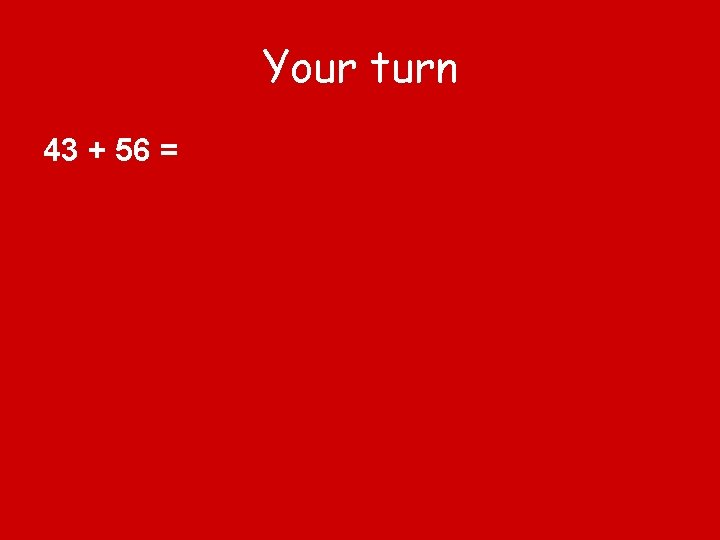Your turn 43 + 56 =Develop methods for adding two digit and three digit numbers by partitioning second number only. 246 + 37= 246 + 30 = 276 + 7 = 282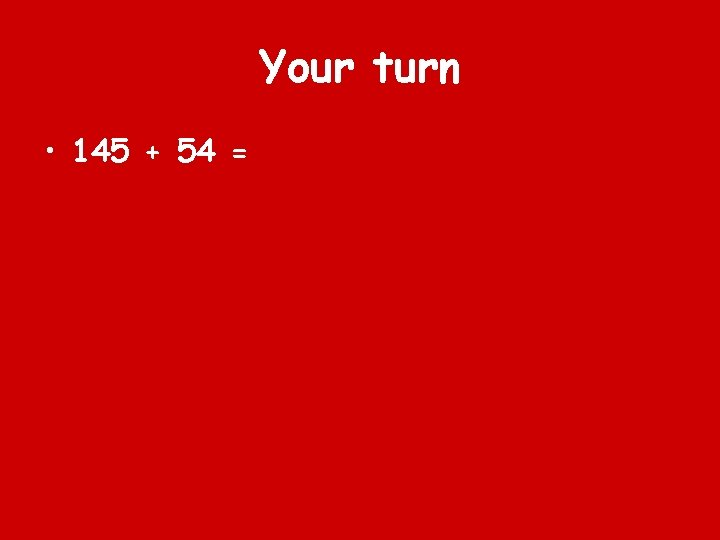Your turn • 145 + 54 =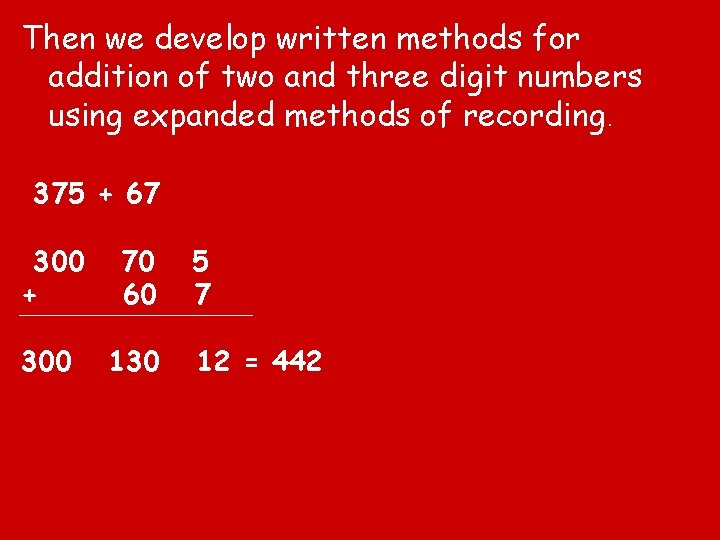Then we develop written methods for addition of two and three digit numbers using expanded methods of recording. 375 + 67 300 + 70 60 300 130 5 7 12 = 442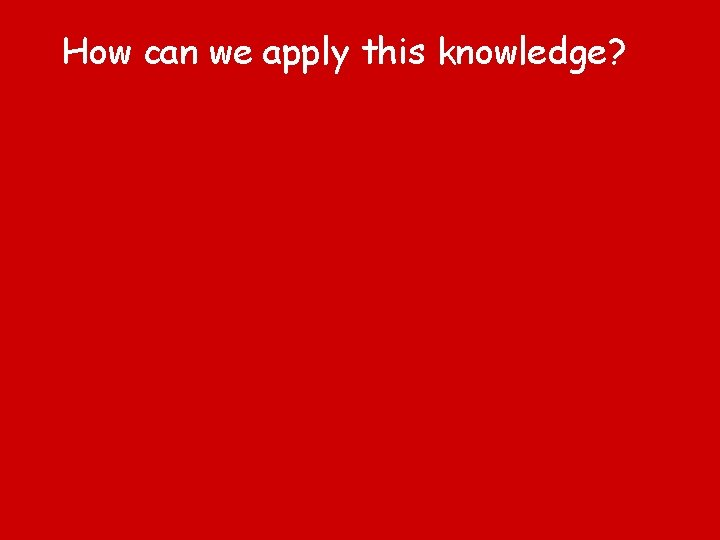How can we apply this knowledge?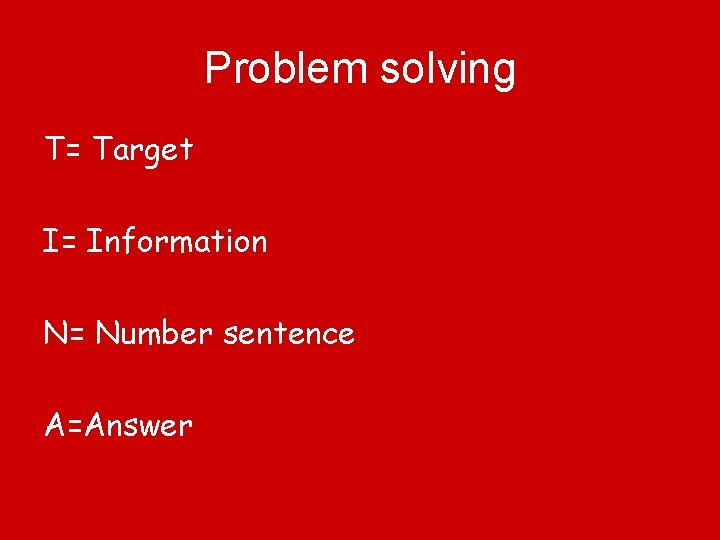Problem solving T= Target I= Information N= Number sentence A=Answer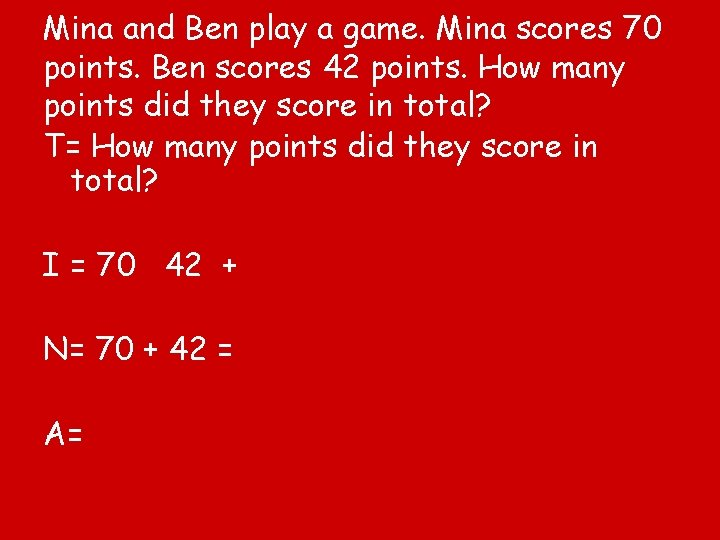Mina and Ben play a game. Mina scores 70 points. Ben scores 42 points. How many points did they score in total? T= How many points did they score in total? I = 70 42 + N= 70 + 42 = A=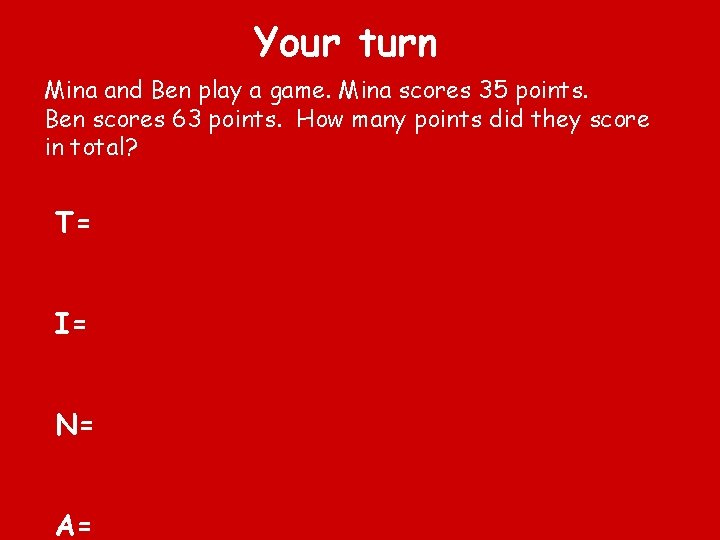Your turn Mina and Ben play a game. Mina scores 35 points. Ben scores 63 points. How many points did they score in total? T= I= N= A=There are 265 children at Hill School. 102 children have a packed lunch. 27 children go home for lunch. The other children have a school lunch. How many children have school lunch?Subtraction Relate subtraction to taking away by counting back and as counting on and record in a number sentence using the – and = signs.Subtraction not crossing through the tens boundary. 46 – 25 = - 25 = 21 In a subtraction calculation we always write the digits of the second number, we don’t draw the image!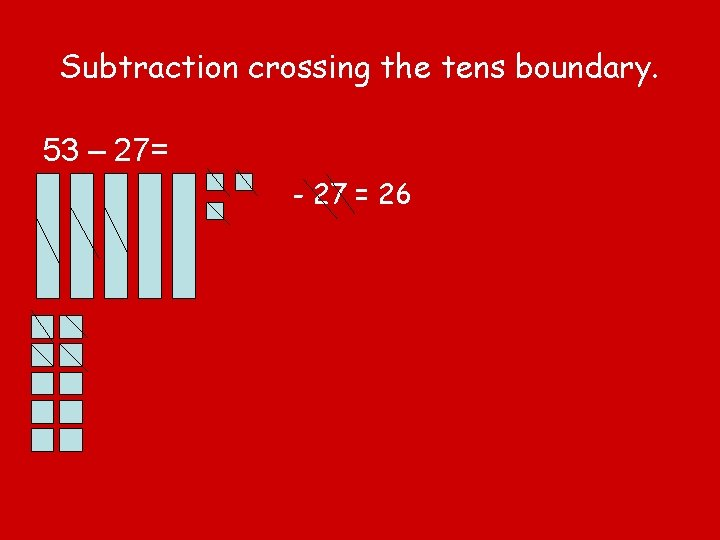Subtraction crossing the tens boundary. 53 – 27= - 27 = 26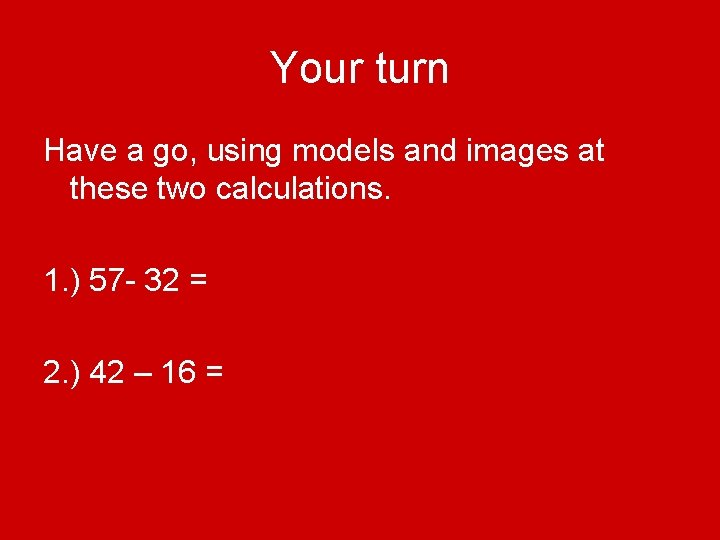Your turn Have a go, using models and images at these two calculations. 1. ) 57 - 32 = 2. ) 42 – 16 =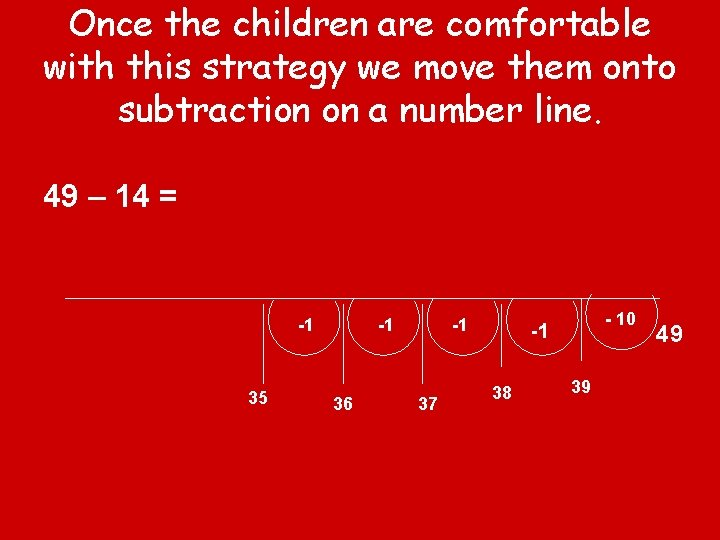Once the children are comfortable with this strategy we move them onto subtraction on a number line. 49 – 14 = -1 35 -1 36 -1 37 - 10 -1 38 39 49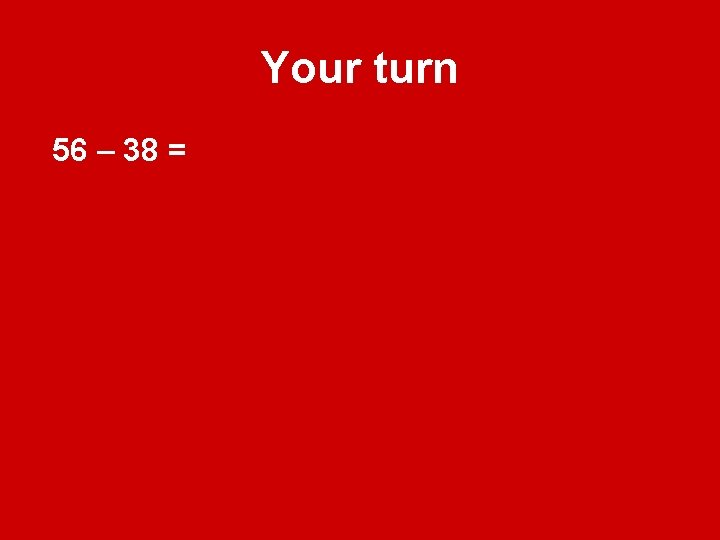Your turn 56 – 38 =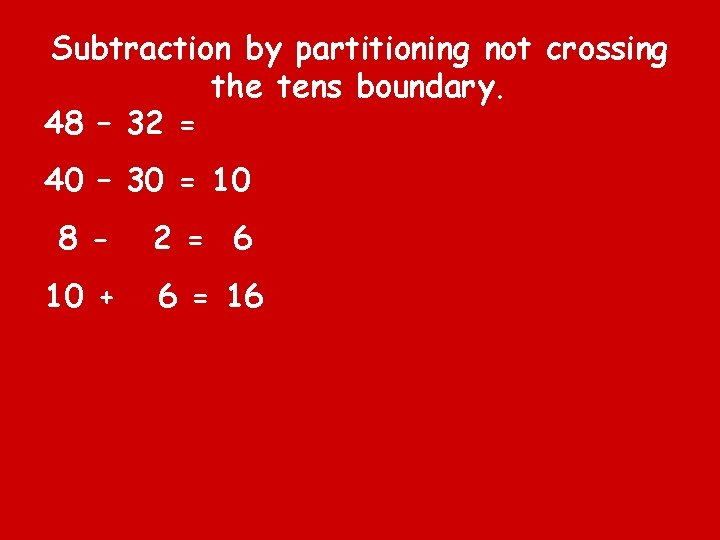Subtraction by partitioning not crossing the tens boundary. 48 – 32 = 40 – 30 = 10 8 - 2 = 6 10 + 6 = 16Subtraction crossing the tens boundary using partitioning. 53 – 37 = 50 – 30 = 20 10 3 - 7= We can’ t do this so we take one of the above tens. 13 – 7 = 6 10 + 6 = 16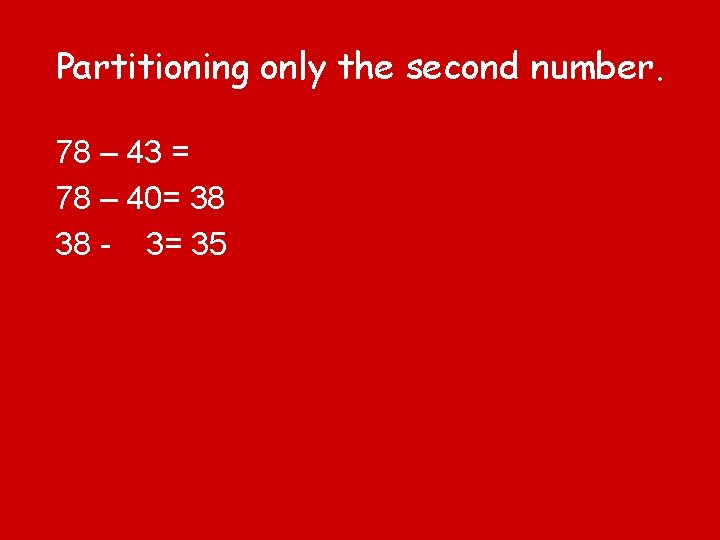Partitioning only the second number. 78 – 43 = 78 – 40= 38 38 - 3= 35This is the same process even when crossing through the tens barrier. 63 - 28 = 63 - 20 = 43 43 - 8= 35Begin to record calculations in preparation for an efficient standard method- expanded decomposition. 81 - 57 70 and 11 50 and 7 20 and 4 = 24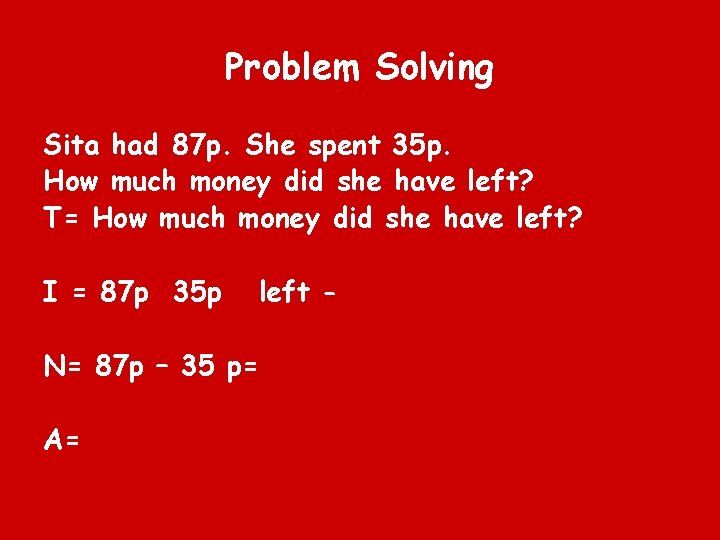Problem Solving Sita had 87 p. She spent 35 p. How much money did she have left? T= How much money did she have left? I = 87 p 35 p left - N= 87 p – 35 p= A=There are 104 children at Delton School. 48 children are girls. How many are boys? T= How many are boys? I= 104 48 - N=104 - 48 A=